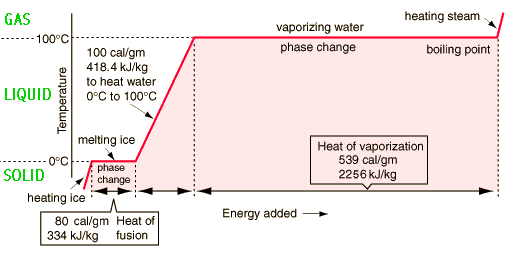# Question c6520

Feb 28, 2015

You'd need about 7 times more energy to vaporize 1 g of water at ${100}^{\circ} \text{C}$ than to melt the same amount of ice at ${0}^{\circ} \text{C}$.

Mathematically, you would know this from the difference between water's latent heat of vaporization and latent heat of fusion.

The latent heat of fusion is the heat required to change a substance from solid state to liquid state; likewise, the latent heat of vaporization is the heat required to change a substance from liquid state to gas state.

Now, here's why you'd need more energy to go from liquid to vapor than you would to go from solid to liquid.

In ice, water molecules are very closely packed together; the intermolecular forces of attraction are particularly strong in solids, which means that the molecules have little to no freedom to move.

When you heat ice at ${0}^{\circ} \text{C}$ to get water at ${0}^{\circ} \text{C}$, you're essentially weakening these intermolecular forces and giving the water molecules freedom of movement. Intermolecular forces still exist, but the water molecules are no longer stuck in a rigid structure.

When going from liquid to vapor, you must provide sufficient heat so that the kinetic energy of the water molecules overcomes the intermolecular forces altogether, leaving you with molecules that no longer interact with eachother.

This being said, the actual values for the latent heat of vaporization and for the latent heat of fusion for water are

DeltaH_("fusion") = "334 J/g"

DeltaH_("vaporization") = "2257 J/g"

The heat you'd need to supply to 1 gram of water in each case would be

q_("fusion") = m * DeltaH_("fusion") = "1 g" * "334 J /g" = "334 J"

q_("vaporization") = m * DeltaH_("vaporization") = "1 g" * "2257 J/g" = "2257 J"#